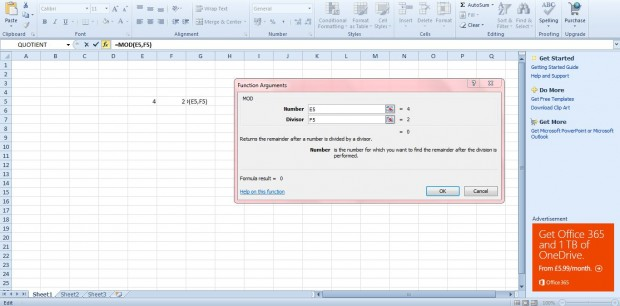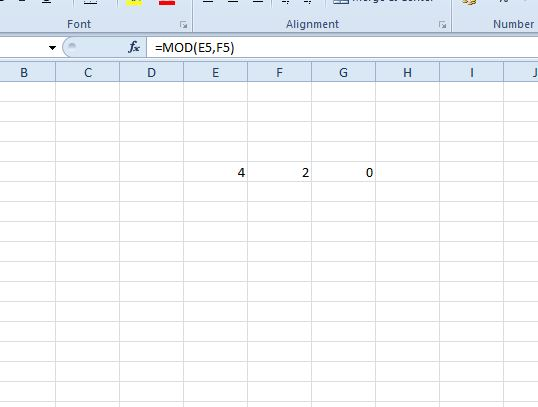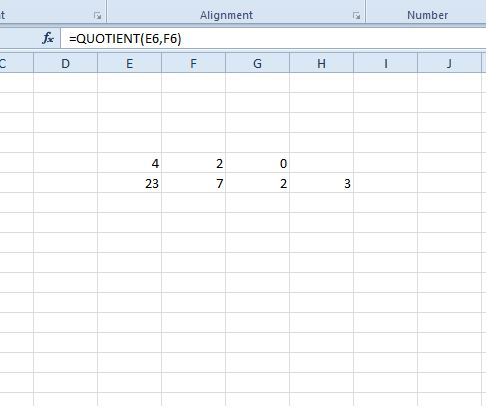# How to use MOD and QUOTIENT functions in Excel spreadsheets [Tip]

MOD and QUOTIENT are two Excel 2010/13 functions you can add to spreadsheets to find the remainder and integer portion of a division between values. These are functions that require just two values. Enter a number and divisor to find the MOD and QUOTIENT.

For example, enter 4 and 2 as a number and divisor in two cells. Click a blank cell, and press fx to open the Insert Function window. Select the MOD function from there to open the window below.Next, press the the button beside the Number field to select the cell with 4 in it. Below that select the cell with 2 in it for the Divisor field. Press OK to add the function to spreadsheet cell.

The MOD cell will include the value 0 as shown in the shot below. As four divides exactly by two there is no remainder. Consequently, 0 is returned as the value. If you had entered 23 and seven as the number and divisor, the MOD value would have been two. Seven divides into 23 three times with a remainder of two.Now let’s try out the QUOTIENT function. This basically shows you the multiple of the divisor. For example, enter 23 and 7 into a couple of spreadsheet cells. Click a cell to add the function to, and select QUOTIENT from the Insert Function window.There select the cell with 23 in it for the Numerator field. Select the cell with 7 for the Denominator value. Click OK to close the window. The QUOTIENT value will be 3. Thus, 3 x 7 amounts to 21 with a MOD remainder of two.